# Disappearing bytes: Reverse engineering the MS Office RTF parser

Microsoft Office was a prime target for attacks in 2017. As well as the large number of vulnerabilities discovered and proof-of-concept exploits published, malware authors felt it necessary to prevent detection of ‘one-day’ and ‘old-day’ exploits by antivirus software. It also became clear that using RTF parsing features and peculiarities are no longer enough to effectively evade detection. Along with the rise of MS Office exploitation, when RTF is used as a container for an exploit, we encountered lots of samples that were ‘exploiting’ the implementation of Microsoft Word’s RTF parser to confuse all other third-party RTF parsers, including those used in AV software.

To achieve parsing exactly like that in MS Office, we needed to reverse-engineer it.

I decided to look first at MS Office 2010, because when it comes to parsing it’s better to look at an older implementation. I then compared my findings with those found in newer versions.

An RTF parser comprises a state machine with 37 states, 22 of which are unique: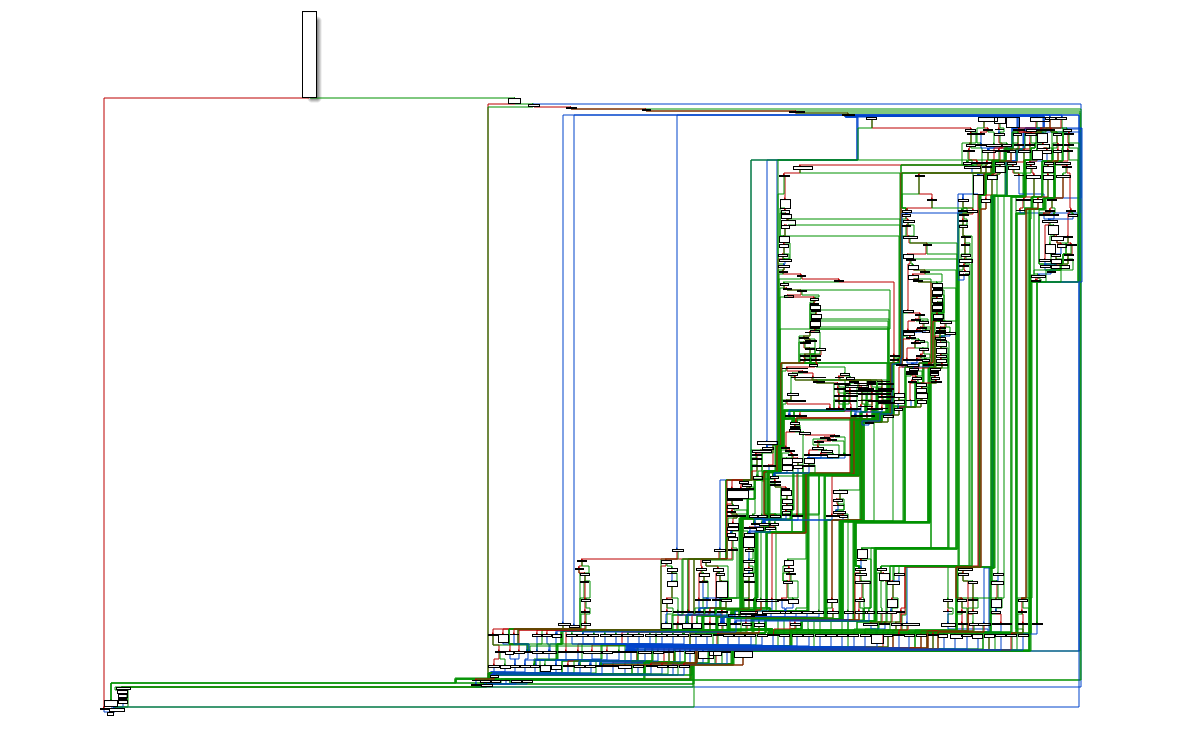We’ll look at the most significant states and those that have an influence on the parsing of objdata, a destination control word that contains the object data. Microsoft OLE links, Microsoft OLE embedded objects, and Macintosh Edition Manager subscriber objects are represented in RTF as objects. These states are:

enum

{

PARSER_BEGIN = 0,

PARSER_CHECK_CONTROL_WORD = 2,

PARSER_PARSE_CONTROL_WORD = 3,

PARSER_PARSE_CONTROL_WORD_NUM_PARAMETER = 4,

PARSER_PARSE_HEX_DATA = 5,

PARSER_PARSE_HEX_NUM_MSB = 7,

PARSER_PARSE_HEX_NUM_LSB = 8,

PARSER_PROCESS_CMD = 0xE,

PARSER_END_GROUP = 0x10,

// …

};

Microsoft Office is shipped without debug symbols, meaning it wasn’t possible to recover the original state names. However, I believe I’ve chosen suitable names according to their underlying functionality.

The first state executed on an opened RTF file is PARSER_BEGIN. In most cases, it’s also executed after processing a control word. The main goal of this state is to determine the next state according to encountered char, destination, and other values stored in the ‘this’ structure and set by control word processors. By default the next state is PARSER_CHECK_CONTROL_WORD.

case PARSER_BEGIN:

// … – checks that we dont need

while (data.pos != data.end)

{

byte = *(uint8_t*)data.pos;

data.pos++;

if (this->bin_size > 0)

{

goto unexpected_char;

}

// …

if (byte == 9)

{

// …

continue;

}

if (byte == 0xA || byte == 0xD)

{

// …

break;

}

if (byte == ‘\’)

{

uint8_t byte1 = *(uint8_t*)data.pos;

if (byte1 == ”’)

{

if (this->destination == listname ||

this->destination == fonttbl ||

this->destination == revtbl ||

this->destination == falt ||

this->destination == leveltext ||

this->destination == levelnumbers ||

this->destination == liststylename ||

this->destination == protusertbl ||

this->destination == lsdlockedexcept)

goto unexpected_char;

state = PARSER_CHECK_CONTROL_WORD;

// …

break;

}

if (byte1 == ‘u’)

{

// …

break;

}

state = PARSER_CHECK_CONTROL_WORD;

// …

break;

}

if (byte == ‘{‘)

{

create_new_group();

// …

break;

}

if (byte == ‘}’)

{

state = PARSER_END_GROUP;

break;

}

unexpected_char:

// it will set next state depending on destination / or go to unexpected_cmd to do more checks and magic

// …

if (this->destination == pict ||

this->destination == objdata ||

this->destination == objalias ||

this->destination == objsect ||

this->destination == datafield ||

this->destination == fontemb ||

this->destination == svb ||

this->destination == macro ||

this->destination == tci ||

this->destination == datastore ||

this->destination == mmconnectstrdata ||

this->destination == mmodsoudldata ||

this->destination == macrosig)

{

state = PARSER_PARSE_HEX_DATA;

data.pos–;

break;

}

// …

break;

}

break;

PARSER_CHECK_CONTROL_WORD will check if the next char is the start of a control word or if it’s a control symbol, and will set the next state accordingly.

case PARSER_CHECK_CONTROL_WORD:

byte = *(uint8_t*)data.pos;

if ((byte >= ‘a’ && byte <= ‘z’) || (byte == ‘ ‘) || (byte >= ‘A’ && byte <= ‘Z’))

{

state = PARSER_PARSE_CONTROL_WORD;

this->cmd_len = 0;

}

else

{

data.pos++;

this->temp = 1;

this->temp = byte;

this->temp = 0;

state = PARSER_PROCESS_CMD;

this->cmd_len = 1;

break;

}

The states PARSER_PARSE_CONTROL_WORD and PARSER_PARSE_CONTROL_WORD_NUM_PARAMETER will store the null-terminated control word that is made up of ASCII alphabetical characters and a null-terminated numeric parameter (if it exists) in a temporary buffer of a fixed size.

case PARSER_PARSE_CONTROL_WORD:

pos = this->temp + 1;

parsed = this->temp + 1;

while (data.pos != data.end)

{

byte = *(uint8_t*)data.pos;

// length of null-terminated strings cmd + num should be <= 0xFF

if ((byte == ‘-‘) || (byte >= ‘0’ && byte <= ‘9’))

{

//if parsed == temp_end

// goto raise_exception

*parsed = 0;

parsed++;

pos = parsed;

if (parsed >= temp_end)

{

parsed = temp_end – 1;

*parsed = 0;

state = PARSER_PROCESS_CMD;

this->cmd_len = pos – (this->temp + 1);

break;

}

data.pos++;

this->cmd_len = pos – (this->temp + 1);

*parsed = byte;

parsed++;

pos = parsed;

state = PARSER_PARSE_CONTROL_WORD_NUM_PARAMETER;

break;

}

if (byte == ‘ ‘)

{

data.pos++;

if (parsed >= temp_end)

{

parsed = temp_end – 1;

}

*parsed = 0;

state = PARSER_PROCESS_CMD;

this->cmd_len = pos – (this->temp + 1);

break;

}

if ((byte >= ‘a’ && byte <= ‘z’) || (byte >= ‘A’ && byte <= ‘Z’))

{

if (parsed – this->temp >= 0xFF)

{

if (parsed >= temp_end)

{

parsed = temp_end – 1;

}

*parsed = 0;

state = PARSER_PROCESS_CMD;

this->cmd_len = pos – (this->temp + 1);

break;

}

//if parsed == temp_end

// goto raise_exception

*parsed = byte;

parsed++;

pos = parsed;

data.pos++;

}

else

{

if (parsed >= temp_end)

{

parsed = temp_end – 1;

}

*parsed = 0;

state = PARSER_PROCESS_CMD;

this->cmd_len = pos – (this->temp + 1);

break;

}

}

break;

case PARSER_PARSE_CONTROL_WORD_NUM_PARAMETER:

while (data.pos != data.end)

{

byte = *(uint8_t*)data.pos;

// length of null-terminated strings cmd + num should be <= 0xFF

if (byte == ‘ ‘)

{

data.pos++;

if (parsed >= temp_end)

{

parsed = temp_end – 1;

}

*parsed = 0;

state = PARSER_PROCESS_CMD;

break;

}

if (byte >= ‘0’ && byte <= ‘9’)

{

if (parsed – this->temp >= 0xFF)

{

if (parsed >= temp_end)

{

parsed = temp_end – 1;

}

*parsed = 0;

state = PARSER_PROCESS_CMD;

break;

}

//if parsed == temp_end

// goto raise_exception

*parsed = byte;

*parsed++;

data.pos++;

}

else

{

if (parsed >= temp_end)

{

parsed = temp_end – 1;

}

*parsed = 0;

state = PARSER_PROCESS_CMD;

break;

}

}

break;

case PARSER_PROCESS_CMD:

case PARSER_SKIP_DATA:

case PARSER_END_GROUP:

case PARSER_SKIP_DATA_CHECK_B:

case PARSER_SKIP_DATA_CHECK_I:

case PARSER_SKIP_DATA_CHECK_N:

case PARSER_SKIP_DATA_GET_BIN_VAL:

case PARSER_SKIP_DATA_INNER_DATA:

this->state = state;

cmd_parser(&data);

state = this->state;

break;

Then it is processed in the state PARSER_PROCESS_CMD that calls another function responsible for processing control words and control symbols. It takes into account the current state and sets the next state.

There are multiple states responsible for parsing hex-data. The most interesting for us is PARSER_PARSE_HEX_DATA – as you can see, it’s set in PARSER_BEGIN if the destination objdata is set.

case PARSER_PARSE_HEX_DATA:

parsed_data = this->temp;

if (this->bin_size <= 0)

{

while (data.pos != data.end)

{

byte = *(uint8_t*)data.pos;

if (byte == ‘{‘ || byte == ‘}’ || byte == ‘\’)

{

state = PARSER_BEGIN;

if (parsed_data != this->temp)

{

push_data(parsed_data – this->temp);

parsed_data = this->temp;

}

break;

}

if (this->flag & 0x4000)

{

data.pos++;

continue;

}

if (byte >= ‘0’ && byte <= ‘9’)

{

val = byte – 0x30;

}

else if (byte >= ‘a’ && byte <= ‘f’)

{

val = byte – 0x57;

}

else if (byte >= ‘A’ && byte <= ‘F’)

{

val = byte – 0x37;

}

else if (byte == 9 || byte == 0xA || byte == 0xD || byte == 0x20)

{

data.pos++;

continue;

}

else

{

// show message that there are not enough memory

this->flag |= 0x4000;

data.pos++;

continue;

}

if (this->flag & 0x8000)

{

this->hex_data_byte = val << 4;

this->flag &= 0x7FFF;

}

else

{

if (parsed_data == temp_end)

{

push_data(sizeof(this->temp));

parsed_data = this->temp;

}

this->hex_data_byte |= val;

*parsed_data = this->hex_data_byte;

parsed_data++;

this->flag |= 0x8000;

}

data.pos++;

}

}

else

{

if (this->flag & 0x4000)

{

uint32_t size;

if (this->bin_size <= data.end – data.pos)

{

size = this->bin_size;

}

else

{

size = data.end – data.pos;

}

this->bin_size -= size;

data.pos += size;

}

else

{

while (this->bin_size > 0)

{

if (parsed_data == temp_end)

{

push_data(sizeof(this->temp));

parsed_data = this->temp;

}

byte = *(uint8_t*)data.pos;

*parsed_data = byte;

parsed_data++;

data.pos++;

this->bin_size–;

}

}

}

if (parsed_data != this->temp)

{

push_data(parsed_data – this->temp);

parsed_data = this->temp;

}

break;

This state will parse hexadecimal data and binary data if set.

The states PARSER_PARSE_HEX_NUM_MSB and PARSER_PARSE_HEX_NUM_LSB are used together to parse hex values (data of the panose control word and control symbol).

case PARSER_PARSE_HEX_NUM_MSB:

this->flag |= 0x8000;

this->hex_num_byte = 0;

state = PARSER_PARSE_HEX_NUM_LSB;

case PARSER_PARSE_HEX_NUM_LSB:

//

byte = *(uint8_t*)data.pos;

data.pos++;

val = 0;

if (byte – ‘0’ <= 9)

{

val = byte – 0x30;

}

else if (byte – ‘a’ <= 5)

{

val = byte – 0x57;

}

else if (byte – ‘A’ <= 5)

{

val = byte – 0x37;

}

this->hex_num_byte |= val << ((this->flag >> 0xF) << 2);

this->flag = ((~this->flag  ^ this->flag) & 0x7FFF) ^ ~this->flag;

if (this->flag & 0x8000)

{

// …

state = PARSER_BEGIN;

}

else

{

break;

}

break;

## State reset

Looking at PARSER_PARSE_HEX_NUM_MSB, PARSER_PARSE_HEX_NUM_LSB and PARSER_PARSE_HEX_DATA, it is easy to spot a bug. Even if they use a different variable to store the decoded hex value, they use the same bit to determine which nibble is now decoded – high (most significant bits, or MSB) or low (less significant bits, or LSB). And PARSER_PARSE_HEX_NUM_MSB always resets this bit to MSB.

It is therefore possible to make bytes disappear in the PARSER_PARSE_HEX_DATA context by triggering a change of state to PARSER_PARSE_HEX_NUM_MSB.

For this to work it is enough to put XX in the data that comes after the objdata control word. In this case, when the parser encounters in state PARSER_PARSE_HEX_DATA it will return to state PARSER_BEGIN and after that will go to state PARSER_PROCESS_CMD. The handler for the control symbol will not change a destination, but will change the next state to PARSER_PARSE_HEX_NUM_MSB. After PARSER_PARSE_HEX_NUM_MSB and PARSER_PARSE_HEX_NUM_LSB control is transferred back to PARSER_BEGIN and eventually to PARSER_PARSE_HEX_DATA because the destination is still equal to objdata. After all that, the next byte will be decoded as a high nibble.

It is also worth noting that PARSER_PARSE_HEX_NUM_LSB does not check if the provided value is a valid hexadecimal; therefore, after there could be absolutely any two bytes.

This behavior can be observed in the following example: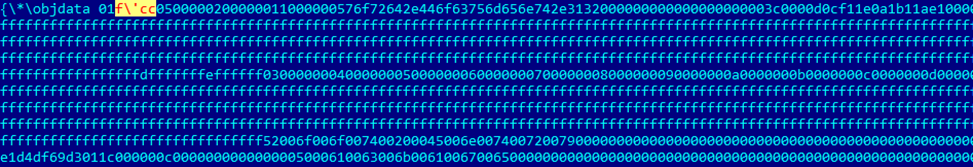“f’cc” will be removed from the final result

When control is transferred for the first time to the PARSER_PARSE_HEX_DATA state, after the objdata control word is processed, the MSB bit is already set. Let’s look at how it happens and how this example will be processed: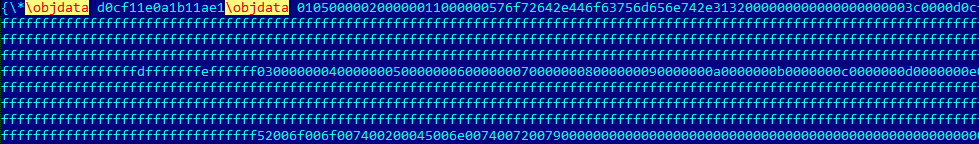After some reverse engineering of the keyword processing function, I found a list of all the control words and their corresponding structures: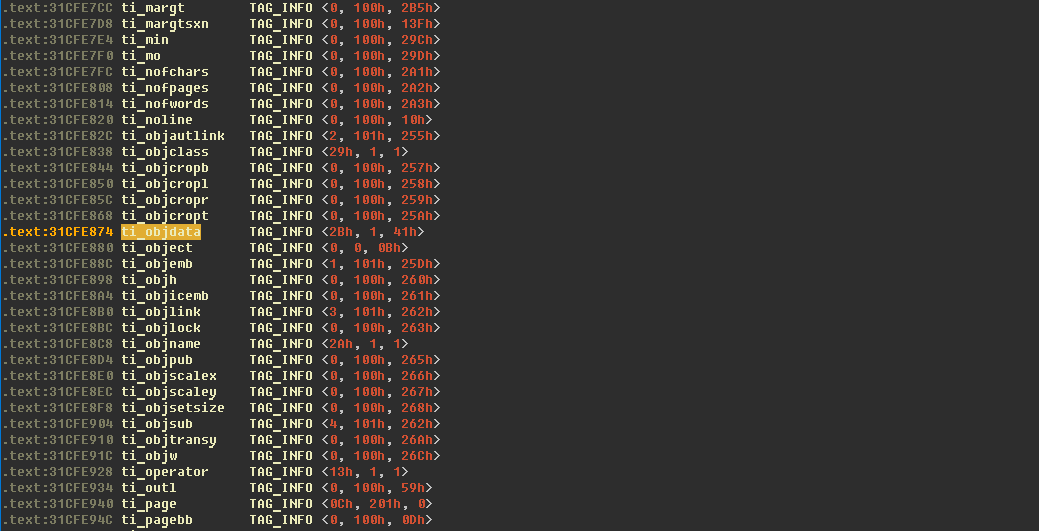With this information we can locate and look at the objdata constructor: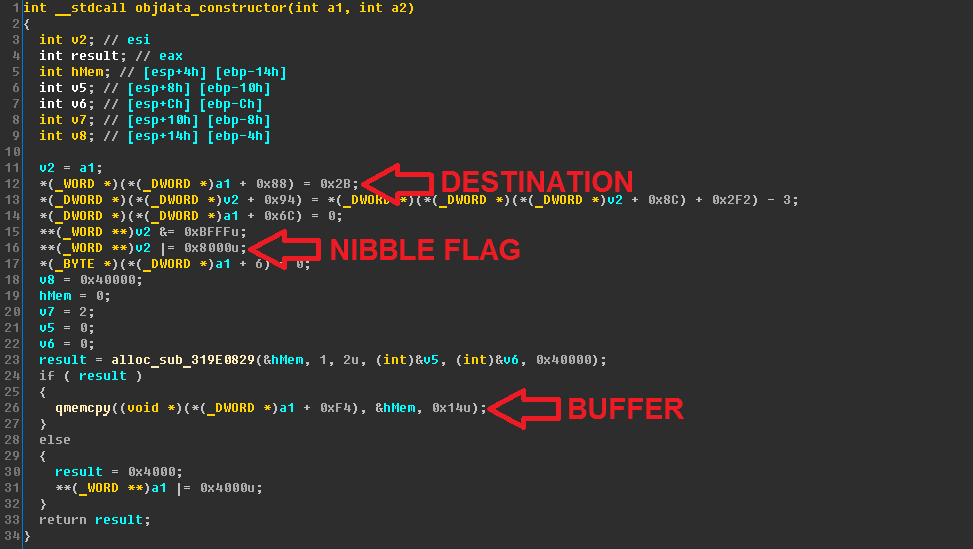You can see it sets the MSB bit, allocates a new buffer and replaces the old pointer with a new one. Therefore, the data decoded between two objdata control words is never used.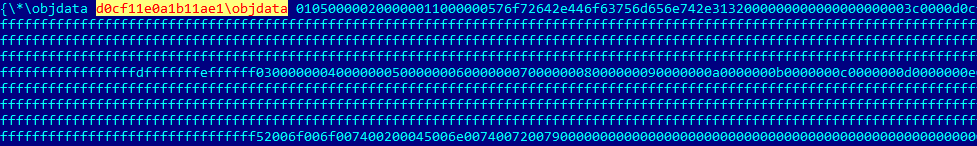“d0cf11e0a1b11ae1” will be removed from the final result

## Final destination

We know that if or objdata is put in data, it will change the output. What about other control words and control symbols? There are more than 1500 of them!

Mostly nothing.

As some control words represent a destination, they can’t be used – they change the objdata destination on their own, and to decode an object the objdata destination is needed.

Other control words do not affect objdata destination.

The only one way to change the destination so that it’s possible to return to the objdata destination without losing previously decoded data is to use special symbols – opening brace ({) and closing brace (}). These symbols indicate the start and end of a group.

When the parser encounters the end of a group in state PARSER_BEGIN, the destination that was set before the start of the group will be restored.

Therefore, by putting {aftncn FF} after objdata, FF will not get into the decoded data because FF now applies to the destination aftncn and will be handled according to this destination.

However, by using {aftnnalc FF}, FF will get into the decoded data because the destination is still equal to objdata.

It is also worth noting that {objdata FF} still can’t be used because the buffer will not be restored.

An accurate list of all destination control words was created with a simple fuzzer.

## Fixed-size buffer

Another obfuscation technique that comes to mind while looking at the code of an RTF parser is not related to this ‘MSB’ bug, but can also be used to remove bytes from a hex-stream. The technique’s related to the temporary buffer size and how a control word and numeric parameter are parsed in the states PARSER_PARSE_CONTROL_WORD and PARSER_PARSE_CONTROL_WORD_NUM_PARAMETER. You can see an example of its use in the following screenshot.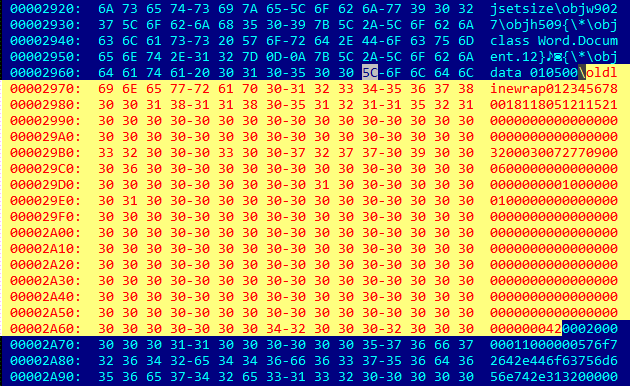In this example the size of the data that will be removed as part of the numeric parameter is calculated using the formula: 0xFF (size of temporary buffer) – 0xB (size of ‘oldlinewrap’) – 2 (null-terminator characters) = 0xF2.

## Unnecessary data

While the techniques described above are related to general RTF parsing, the processing of some specific keywords conceals some further confusion.

According to the specification states, if * was encountered right before a control word or control symbol that was not found in the lookup table, its considered an unknown destination group and all the data up to the closing brace } that closes this group should be discarded. The lookup table in MS Office contains control words that are not present in the specification and it raises concerns that it will be changed in future, affecting parsing of the same document on different versions of MS Office. When the function responsible for processing keywords encounters such cases or one of the specific control words (such as comment, generator, nonshppict and so on), it will set the state PARSER_SKIP_DATA and the number for encountered opening braces { to 1.

enum

{

// …

PARSER_SKIP_DATA = 0xF,

// …

PARSER_SKIP_DATA_CHECK_B = 0x13,

PARSER_SKIP_DATA_CHECK_I = 0x14,

PARSER_SKIP_DATA_CHECK_N = 0x15,

PARSER_SKIP_DATA_GET_BIN_VAL = 0x16,

PARSER_SKIP_DATA_INNER_DATA = 0x17,

// …

};

## Kind of magic

During analysis of the PARSER_SKIP_DATA* states I found things that are the opposite not only to the specification but also to the rest of the parser code.

While looking for the bin control word, this states will skip data, changing the number of encountered opening and closing braces until that number equals zero. The hidden catch lies in the way the numeric parameter is processed.

First of all, the maximum allowed length of the numeric parameter is increased up to 0xFF – it’s calculated without considering the length of the control word.

The second catch is that the numeric parameter is not numeric anymore! The parser allows not only decimal characters but also Latin characters to pass. Then this parameter is passed to custom strtol, making it possible to specify the length of data that should be skipped without considering opening and closing braces as a hexadecimal number.

Obfuscations with the use of these two primitives have not yet been encountered in the wild.

## Conclusion

Reverse engineering has proved to be the most effective way to build a parser, and in the case of RTF it would most likely be impossible to achieve the desired behavior otherwise.

Exact parsing depends on small implementation details and algorithmic bugs rather than on a specification that could be confusing or state things that are not true.

Kaspersky Lab products detect all kinds of RTF obfuscation and perform the most correct processing of RTF files, providing the best protection to our end users.

### Related Posts

1. pyFlorist

Amazing as usual. Keep more coming.

2. somsai rune

hello sir,
which office dll you use for reversing the rft parser.
I want to know which dll you open in ida pro to find the rtf parser graph.